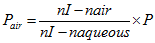# SRK formula

## SRK formula

An approximate formula established by Sanders, Retzlaff and Kraft to determine the power of an intraocular lens implant P, in aqueous, to render the eye emmetropic (and ignoring the lens thickness)
P = A − 2.5X − 0.9K
where A is a numerical term specific to the implant and to the manufacturer. It is equal, on average, to 115 for anterior chamber implants and 116.8 for posterior chamber implants. X is the axial length of the eye (in mm) and K is the average keratometer reading (in dioptres). The formula gives satisfactory results for eyes of average length. Example: A = 116.8, X = 23mm and K = 43D, P = 116.8 − 57.5 − 38.7 = 20.6D.The power of the intraocular lens implant differs in air and is given by the following formulawhere nI is the index of refraction of the implant and P its power in aqueous. Example: assuming nI is equal to 1.523 and the power is 20.6D, Pair = (1.523 − 1.0)/(1.523 −1.333) ✕ 20.6 = 56.70D.There are several other formulae used to predict the power of an intraocular lens implant (e.g. Holladay, Hoffer Q). See biometry of the eye; intraocular lens.
Millodot: Dictionary of Optometry and Visual Science, 7th edition. © 2009 Butterworth-Heinemann
Site: Follow: Share:
Open / Close